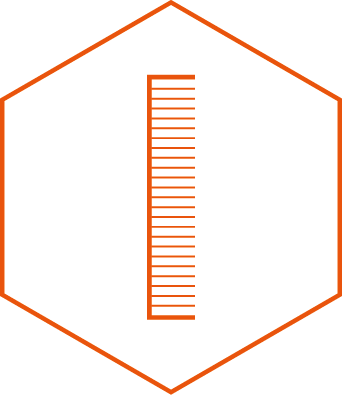# Sizes and Measurements

One Acre = 4,840 Square Yards
One Hectare = 10,000 Square Metres

## To convert :- Linear Measurement

Hectares to Acres Multiply by 2.260

Acres to Hectares Multiply by 0.442

Yards to Metres Multiply by 0.914

Metres to Yards Multiply by 1.094

Feet to Metres Multiply by 0.305

Metres to Feet Multiply by 3.281

## To Convert :- Area Measurement

Square Yards to Square Metres Multiply by 0.836

Square Feet to Square Metres Multiply by 0.093

Square Metres to Square Yards Multiply by 1.196

Square Metres to Square Feet Multiply by 10.764

Quarter Acre = 1011 Square Metres (Approx 50 metres x 20 metres) 10,890 Square Feet (Approx 150 feet by 72 feet)

Third of an Acre = 1348 Square Metres (Approx 60 metres x 22 metres) 14,517 Square Feet (Approx 180 Feet by 80 feet)

Half Acre = 2023 Square Metres (Approx 70 metres x 30 metres) 21,780 Square Feet (Approx 240 feet by 90 feet)View our Land Size Examples guide